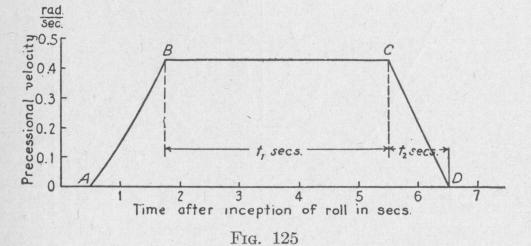Previous Page Index(For best results view this page as a PDF) Next Page

158   ANTI-ROLL DEVICES FOR SHIPS

This value is slightly different from that obtained from the assumption in (108). Our guess of one degree displacement during the first quarter-second was too high. The displacement was more nearly 0.55°. If now we go through a computation as above with 0.55 as the assumed value of 0', we shall find that this value will be checked very closely. This is the value to be used.

In the same manner we find values for instantaneous precessional velocity at the end of each quarter-second interval during the first 1.25 sec. of precession, the angular displacement of the gyro-axle from the end of an oscillation and from the equilibrium position at the end of each quarter-second interval, and the mean angular displacement during these quarter-second intervals. The values found are given in columns 5, 6, 7, and 8 of the table.

After 1.25 sec. from the beginning of precession, the brake is applied so as to maintain the precessional velocity constant till the instant at which the velocity is to be given a constant deceleration sufficient to bring the gyro-axle to the end of the 120° swing in 6.5 sec. from the time it was at the other end of the swing. While the velocity of precession is constant, that is, from tp = 1.25 sec. till the instant when the final rapid deceleration is started, values of (p', 01, and 4' can be obtained from the equations:

¢' _ oil + wpt 57.3° (110) ,ps = 60° - p' degree (111) 4) = 60° - 2 (0,' + 02') degree   (112)

For example, when tp = 1.25 sec.:

14.78° + 0.4333(0.25)57.3 = 20.99°

¢i = 60° - 20.99° = 39.01° = 39° 1'

4 = 60 - 2 (20.99° + 14.78°) = 42.12° = 42° 20'

(e) The Time at Which the Final Deceleration of the Precessional Velocity of the Gyro-Axle Should Begin. - As stated in the problem, the angular velocity of the gyro-axle 1.25 sec. after leaving one end of a swing is to be maintained constant until the gyro-axle has come to near the other end of the swing. When the gyro-axle has rotated for the proper time, the brake effect is suddenly increased, thereby bringing the velocity to zero at the end of the 6.5-sec. halfperiod. We shall now determine the number of seconds after the inception of precession, when a constant deceleration must begin in order that the gyro-axle may attain a displacement of 120° from the other end of the swing in 6.5 sec.

From column 5 of the table (p. 161), we see that 1.25 sec. after the inception of precession, or 1.75 sec. after the inception of the roll, the angular velocity of the gyro-axle is 0.4333 rad. per sec. This is represented by the point B, Fig. 125. Suppose that the constant deceleration must begin at some point C, which is t, sec. later than B. Represent by t2 the time interval from C to the end of the 6.5-sec. half-cycle at D, when the gyro-axle has traversed 120° to this end of a swing. Then, reckoning time from the inception of the roll:

ti + t2 = (6.5 - 1.75) sec. = 4.75 sec. t, = 4.75 sec. - t2

THE ACTIVE TYPE OF GYRO SHIP STABILIZER 159

From column 6 of the table (p. 161), the angle through which the gyro-axle moves during the time t, + t2 is

120.0° - 14.78° = 105.22° = 1.836 rad.

Since the deceleration is uniform, the mean velocity of precession during the time t2 is 2 (0.4333) rad. per sec. Hence,

1.836 rad. = (0.4333), + 2 (0.4333)t2

= (0.4333)(4.75 - t2) + (0.2166)t2 = 2.058 - 0.2166 t2

Consequently,

t2 - (2.058 - 1.836) rad. = 1.0 sec. 0.2166 rad. per sec.

Therefore, the final deceleration of the precession of the gyro-axle must be started (6.5 - 1.0) sec. = 5.5 sec. after the inception of the roll or 5.0 sec. after the inception of precession.

If t2 comes out with a negative sign, we would know that with the present constant angular velocity of the gyro-axle during the middle part of a halfcycle, the gyro-axle would need to precess for longer than the half-period before it would reach the end of an oscillation. In this case we would need to increase the velocity during the middle of the half-cycle by allowing the initial acceleration to last for a longer time than that allowed in the problem. Again, if t2 is positive, but so small that the final deceleration would need to be completed in too short a time, then an impracticable brake torque may be required. In this case, also, we would allow the initial acceleration to last for a fraction of a second longer than that previously allowed.

The value of t, should be from 0.5 sec. to 1.0 sec. For a gyro-wheel of the size here used, the value of t2 we have obtained is larger than strictly necessary. We could have stopped the initial acceleration a little sooner.

(f) The Values of the Gyroscopic Torque Opposing Roll at the End of Each Half-Second During One Half-Cycle of Roll. - When the precessing gyro-axle is in the equilibrium position, the torque exercised by the gyroscope on the ship is

Ksw,wp] = Ks' 2 2rn   - (333350)1310

Lg[=   wp = 1422400 wp

a~ iFn wp   anvPrevious Page Index(For best results view this page as a PDF) Next Page